February 13, 2017

# Extending KeLP

A new representation can be added by creating a class that implements Representation.
The programmer will be asked for implementing two specific methods, getTextFromData and setDataFromText, which are necessary for serializing and deserializing the Representation in a string format and deserializing it. The programmer can decide the textual format describing the new representation. This means that a specific JSON format is not imposed. For instance, we use the text format of libSVM for sparse vectors and the Penn Treebank notation for the tree representation, as shown in the page, Input Data Format.
An empty constructor must be included. Optionally, the class can be annotated with @JsonTypeName in order to specify an alternative type name to be used during the serialization/deserialization mechanism, otherwise the class name will be automatically used.
If a normalization function need to be defined on the representation (e.g., the representation is a vector, a matrix or a higher order tensor), then the Normalizable interface can be implemented, enabling some useful preprocessing operations on datasets such as feature scaling or data normalization. In this case the following methods must be implemented:

• getSquaredNorm: returns the squared norm of this vector
• normalize: scales the representation in order to have a unit norm in the explicit feature space
• scale: multiplies each element of the representation by a given coefficient

As discussed in Kernels, kernels are organized into four main abstractions, i.e., DirectKernel, KernelComposition, KernelCombination, and KernelOnPairs. Therefore, when implementing a new kernel, the first step is understanding which abstract class we must extends. In this guide, we will describe how to implement a new DirectKernel and a new KernelComposition; the extensions to the other kernel types is straightforward.

In describing how to implement a new Kernel we will use the a linear kernel and a radial basis function (RBF) kernel as practical examples.

### IMPLEMENTING A DIRECT KERNEL: THE LINEAR KERNEL EXAMPLE

The linear kernel is simply the dot product between two vectors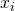and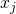, i.e.,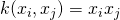. Then, in implementing LinearKernel, we need to extend a DirectKernel on Vector. Optionally the class can be annotated with @JsonTypeName in order to specify an alternative type name to be used during the serialization/deserialization mechanism.

To make the JSON serialization/deserialization mechanism work, an empty constructor must be defined for the LinearKernel:

Finally the kernelComputation method must be implemented:

### IMPLEMENTING A KERNEL COMPOSITION: THE RBF KERNEL EXAMPLE

The RBF kernel function is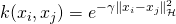where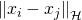is the distance between two examplesandin a Reproducing Kernel Hilbert Space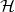. Considering a generic RKHS, the simple euclidean distance in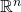can be generalized in order to define a distance measure applicable to any underlying kernel, operating on any representation. Then RbfKernel must extend KernelComposition. Optionally, the class can be annotated with @JsonTypeName in order to specify an alternative type name to be used during the serialization/deserialization mechanism.

To make the JSON serialization/deserialization mechanism work, an empty constructor must be defined for the RbfKernel, and all the kernel parameters must be associated with the corresponding getter and setter methods. In this case gamma is the only parameter to be serialized and the corresponding getGamma and setGamma methods must be implemented.

Finally the kernelComputation method must be implemented containing the specific kernel similarity logic.

# Adding a New Learning Algorithm

As discussed in Learning Algorithms, KeLP provides several learning algorithms, which can be used when implementing new others. In this guide, we describe the implementation of a new linear algorithm for binary classification. A similar procedure can be easily used to implement other different types of learning algorithms.

### IMPLEMENTING NEW LEARNING ALGORITHMS: THE PEGASOS EXAMPLE

We are now pretending that the PegasosLearningAlgorithm is not available in KeLP, and that we need to implement it from scratch.
Pegasos (Shalev-Shwartz et al., 2007) is an efficient solver for linear SVM for binary classification tasks that uses a mini-batch approach; therefore, we need to implement ClassificationLearningAlgorithm, BinaryLearningAlgorithm and LinearMethod. Optionally, the class can be annotated with @JsonTypeName in order to specify an alternative name to be used during the JSON/XML serialization/deserialization mechanism.

KeLP decouples the learning from the prediction phase. Regarding PegasosLearningAlgorithm, the prediction function is common to other classification algorithms. Thus, it is possible to use the class BinaryLinearClassifier, which is already implemented in the platform. However, we need to add a new corresponding parameter, i.e., classifier. Thus, we need to add an empty constructor along with all the learning parameters associated with the corresponding getter and setter methods. These are required by the JSON serialization/deserialization mechanism. The learning parameters of Pegasos are the regularization coefficient lambda, the number of iterations T and the number of examples k exploited during each mini-batch iteration.

According to the selected interfaces some specific methods have to be implemented. As any LinearMethod we need to implement setRepresentation and getRepresentation which refer to the String identifier for the specific Representation object the algorithm must exploit. Obviously a corresponding parameter must created, i.e., representation

As any BinaryLearningAlgorithm we need to implement getLabel and setLabel which refer to the Label that must considered as positive class. Obviously a corresponding parameter must created, i.e. label. Moreover, to be compliant with the LearningAlgorithm interface, the methods getLabels and setLabels must be implemented. For the special case of BinaryLearningAlgorithm they must operate on a single entry List:

Finally, as any ClassificationLearningAlgorithm we need to implement:

• getPredictionFunction: the proper prediction function must be returned, in this case the classifier object. It is a good practice to specialize the returning type, i.e., the method must return a BinaryLinearClassifier instead of a generic Classifier;
• duplicate: the instance of an algorithm must be created and returned, copying all the learning parameters (the state variables must not be set to their default value). It is a good practice to specialize the returning type, i.e., the method must return a PegasosLearningAlgorithm instead of a generic LearningAlgorithm;
• reset: it forces the algorithm to it default state, forgetting the learning process already conducted;
• learn: this method must implement the learning process of Pegasos.

### References

S. Shalev-Shwartz, Y. Singer, and N. Srebro. Pegasos: Primal estimated sub–gradient solver for SVM. In Proceedings of the International Conference on Machine Learning, 2007.